# 【c51单片机】交通红绿灯设计

1、初始时：南北向、东西向直行左转均为红灯

2、南北向直行：

①南北向直行绿灯亮，延时若干秒

②启动南北向左转数码管显示：倒计时6秒；同时南北向直行绿灯闪烁3下，灭亮灭亮灭亮共3秒，然后南北向直行绿灯灭，南北向直行黄灯亮，延时3秒

③南北向左转数码管倒计时显示结束，关闭；同时南北向直行黄灯灭，南北向直行红灯亮

3、南北向左转：

①南北向左转绿灯亮，延时若干秒

②启动东西向直行数码管显示：倒计时6秒；同时南北向左转绿灯闪烁3下，灭亮灭亮灭亮共3秒，然后南北向左转绿灯灭，南北向左转黄灯亮，延时3秒

③东西向直行数码管倒计时显示结束，关闭；同时南北向左转黄灯灭，南北向左转红灯亮

4、东西向直行：

①东西向直行绿灯亮，延时若干秒

②启动东西向左转数码管显示：倒计时6秒；同时东西向直行绿灯闪烁3下，灭亮灭亮灭亮共3秒，然后东西向直行绿灯灭，东西向直行黄灯亮，延时3秒

③东西向左转数码管倒计时显示结束，关闭；同时东西向直行黄灯灭，东西向直行红灯亮

5、东西向左转：

①东西向左转绿灯亮，延时若干秒

②启动南北向直行数码管显示：倒计时6秒；同时东西向左转绿灯闪烁3下，灭亮灭亮灭亮共3秒，然后东西向左转绿灯灭，东西向左转黄灯亮，延时3秒

③南北向直行数码管倒计时显示结束，关闭；同时东西向左转黄灯灭，东西向左转红灯亮

1、初始时：南北向、东西向直行左转均为红灯

2、南北向直行：

①南北向直行绿灯亮，延时若干秒

②启动南北向左转数码管显示：倒计时6秒；同时南北向直行绿灯闪烁3下，灭亮灭亮灭亮共3秒，然后南北向直行绿灯灭，南北向直行黄灯亮，延时3秒

③南北向左转数码管倒计时显示结束，关闭；同时南北向直行黄灯灭，南北向直行红灯亮

3、南北向左转：

①南北向左转绿灯亮，延时若干秒

②启动东西向直行数码管显示：倒计时6秒；同时南北向左转绿灯闪烁3下，灭亮灭亮灭亮共3秒，然后南北向左转绿灯灭，南北向左转黄灯亮，延时3秒

③东西向直行数码管倒计时显示结束，关闭；同时南北向左转黄灯灭，南北向左转红灯亮

4、东西向直行：

①东西向直行绿灯亮，延时若干秒

②启动东西向左转数码管显示：倒计时6秒；同时东西向直行绿灯闪烁3下，灭亮灭亮灭亮共3秒，然后东西向直行绿灯灭，东西向直行黄灯亮，延时3秒

③东西向左转数码管倒计时显示结束，关闭；同时东西向直行黄灯灭，东西向直行红灯亮

5、东西向左转：

①东西向左转绿灯亮，延时若干秒

②启动南北向直行数码管显示：倒计时6秒；同时东西向左转绿灯闪烁3下，灭亮灭亮灭亮共3秒，然后东西向左转绿灯灭，东西向左转黄灯亮，延时3秒

③南北向直行数码管倒计时显示结束，关闭；同时东西向左转黄灯灭，东西向左转红灯亮

•  PROTEUS元件表
 元件名称 型号 数量 Proteus关键字 单片机 AT89C51 1 AT89C51 晶振 11.0592MHz 1 CRYSTAL 电容 22pF 2 CAP 电解电容 10uF 1 CAP-ELEC 按键 1 BUTTON 电阻 220Ω 1 RES 电阻 10kΩ 1 RES 电阻 700Ω 1 RES 排阻 800Ω 1 RESPACK 数码管 8 7SEG 交通灯 8 TRAFFIC 锁存器 2 74HC573
• 数码管、交通灯、锁存器查找方式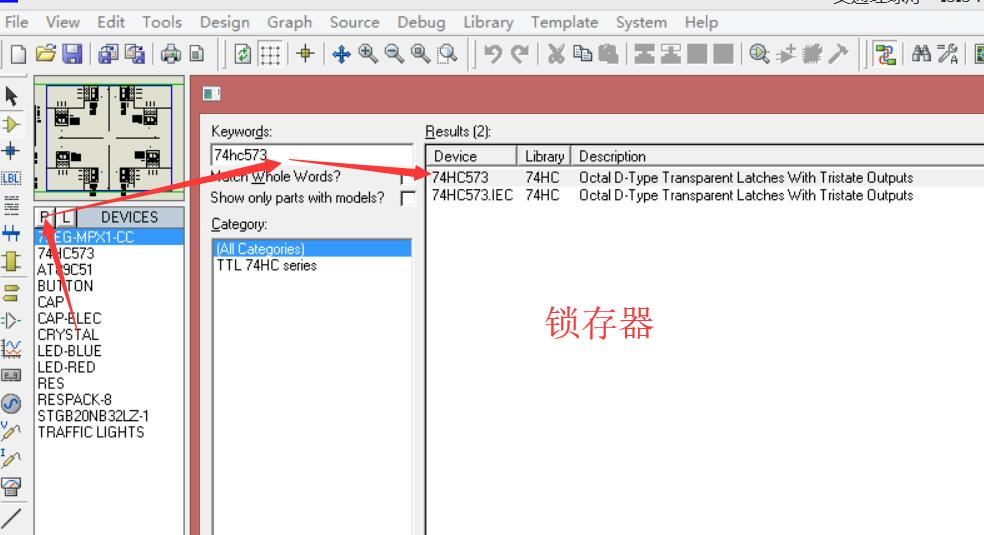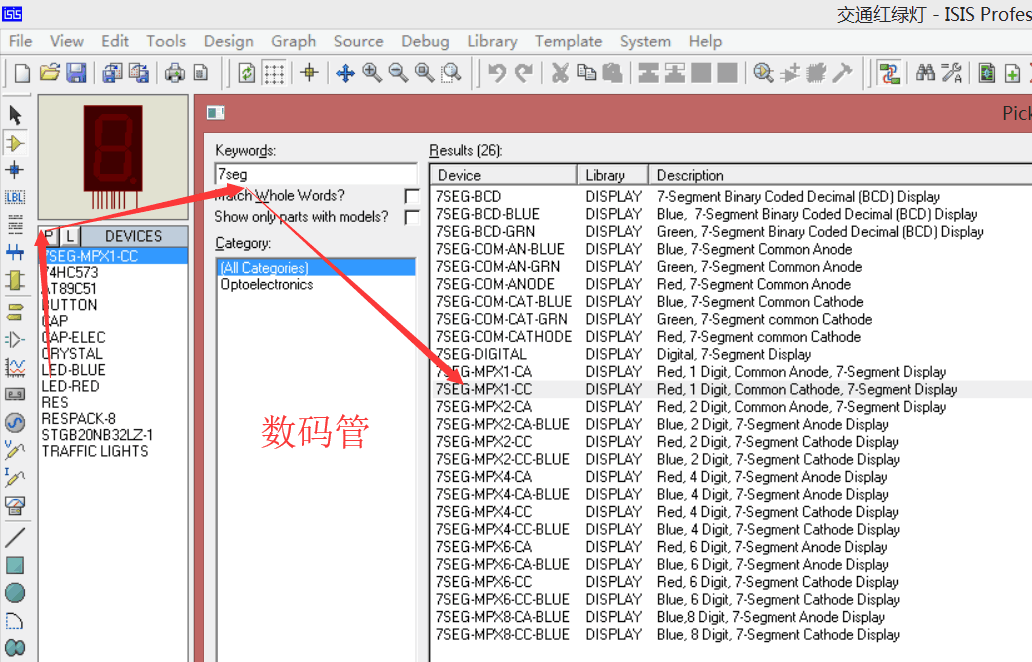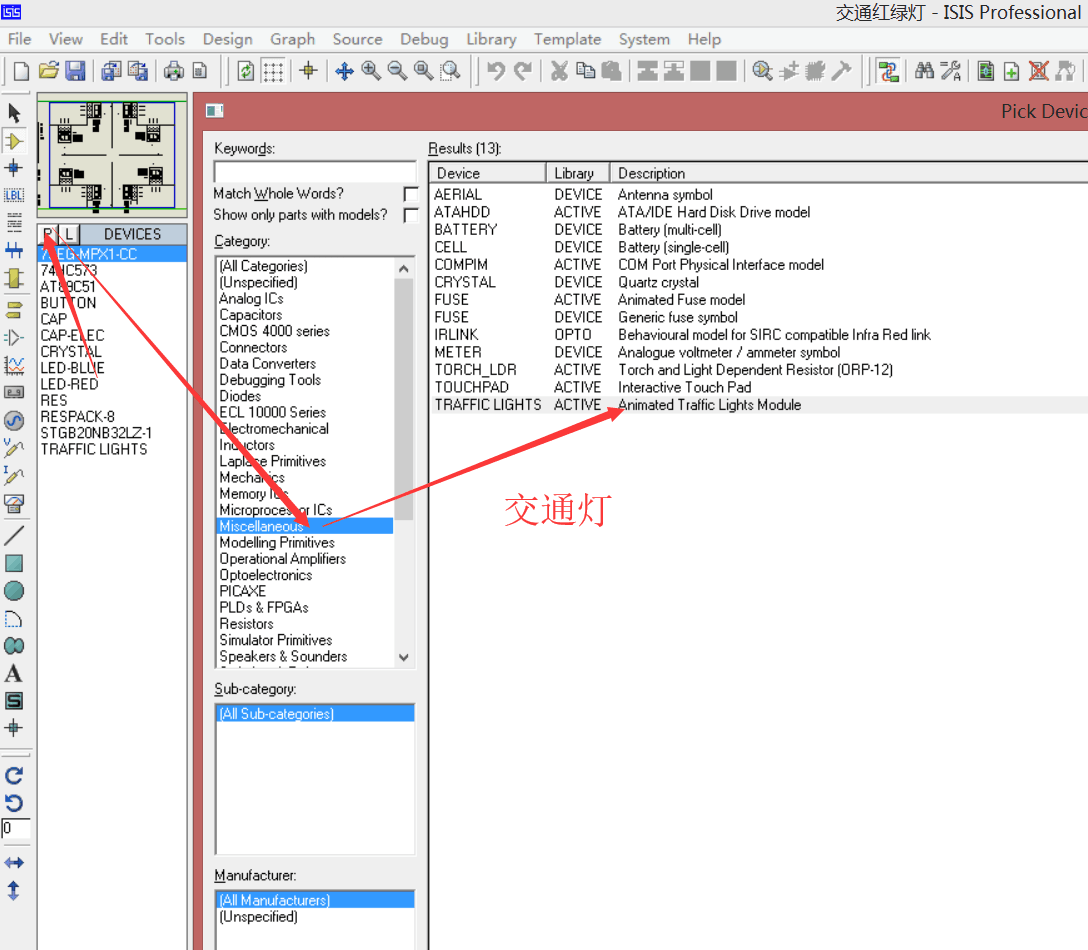• 在PROTEUS中设计的单片机系统电路图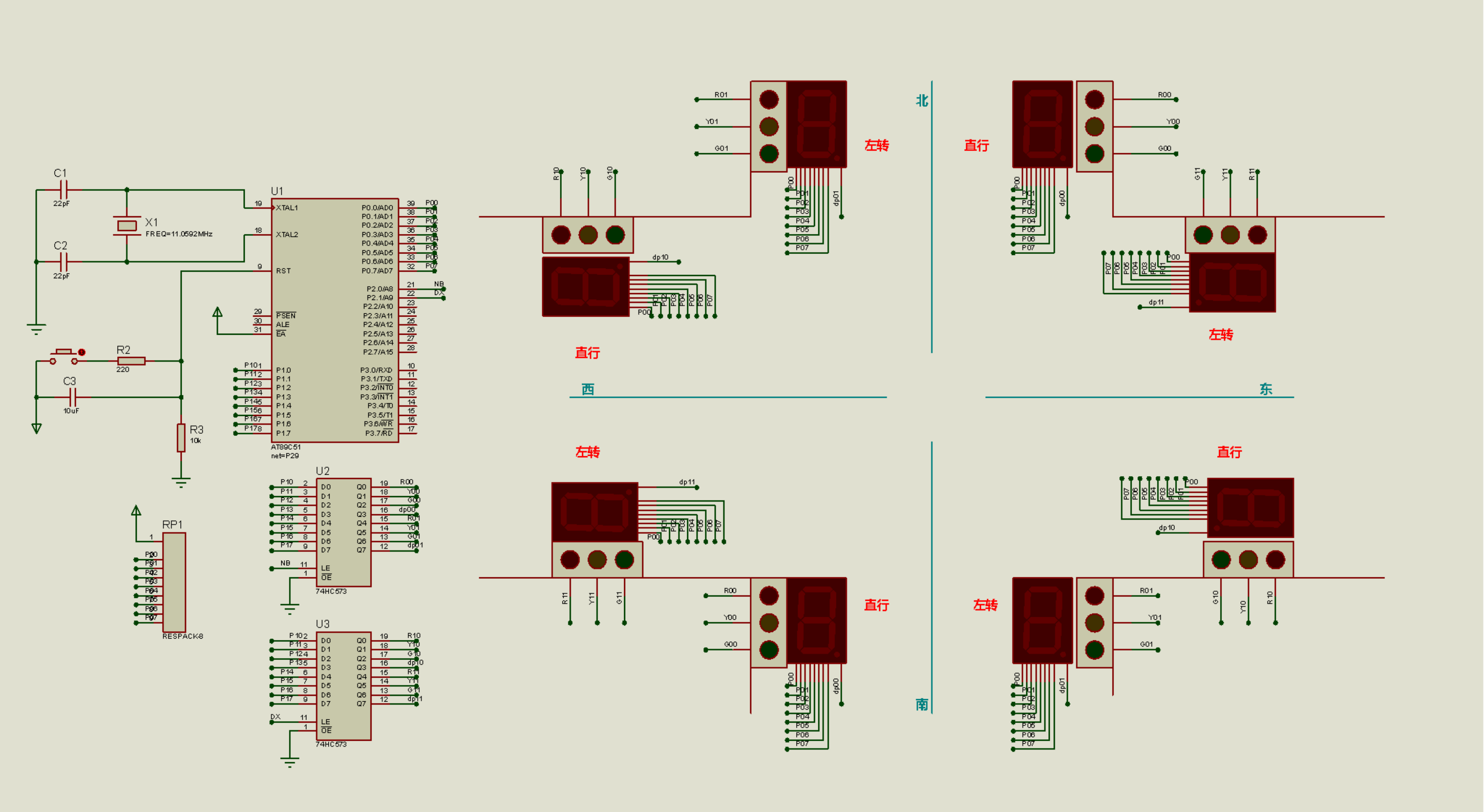• 在KEIL中编写的源程序

/***********************
作者：申俊
2018.10.4
**************************/
#include <reg51.h>
unsigned char code table[]={
0x3f,0x06,0x5b,0x4f,
0x66,0x6d,0x7d,0x07,
0x7f,0x6f};
void delay(int t)
{int i,j;
for(i=0;i<=t;i++)
for(j=0;j<=110;j++);
}
sbit NB=P2^0;//南北，低4位控制直行，高四位控制左转向
sbit DX=P2^1;//东西，低4位控制直行，高四位控制左转向
int i;
void main()
{
NB=1;P1=0X88;NB=0;
DX=1;P1=0X88;DX=0;
P0=0X00;

/*1、南北向、东西向直行左转均为红灯*/
NB=1;P1=0X99;NB=0;//南北直、左红灯亮
DX=1;P1=0X99;DX=0;//东西直、左红灯亮
delay(3000);
NB=1;P1=0X88;NB=0;//南北直、左红灯灭
DX=1;P1=0X88;DX=0;//东西直、左红灯灭

/*2、南北向直行(NB)*/
NB=1;P1=0X84;NB=0;//南北直绿灯亮
delay(3000);
//南北向左转数码管显示：倒计时6秒
for(i=6;i>=4;i--) //南北向直行绿灯闪烁3下
{
NB=1;P1=0X0C;NB=0;//南北直绿灯亮
P0=table[i];
delay(500);
NB=1;P1=0X08;NB=0;//南北直绿灯灭
delay(500);
}
NB=1;P1=0X0C;NB=0;//南北直绿灯亮
for(i=3;i>=0;i--)
{
P0=table[i];
delay(1000);
}
NB=1;P1=0X8A;NB=0;//南北直黄灯亮
delay(3000);
NB=1;P1=0X88;NB=0;//南北直黄灯灭,左转数码管倒计时显示结束

/*3、南北向左转(NB)*/
NB=1;P1=0X48;NB=0;//南北左绿灯亮
delay(3000);
//南北向直行数码管显示：倒计时6秒
for(i=6;i>=4;i--) //南北向左转绿灯闪烁3下
{
NB=1;P1=0XC0;NB=0;//南北左转绿灯亮
P0=table[i];
delay(500);
NB=1;P1=0X80;NB=0;//南北左转绿灯灭
delay(500);
}
NB=1;P1=0XC0;NB=0;//南北左转绿灯亮
for(i=3;i>=0;i--)
{
P0=table[i];
delay(1000);
}
NB=1;P1=0XA8;NB=0;//南北左转黄灯亮
delay(3000);
NB=1;P1=0X88;NB=0;//南北左转黄灯灭,直行数码管倒计时显示结束
delay(1000);
NB=1;P1=0X99;NB=0;

/*4、东西向直行(NB)*/
DX=1;P1=0X84;DX=0;//东西北直绿灯亮
delay(3000);
//东西向左转数码管显示：倒计时6秒
for(i=6;i>=4;i--) //东西向直行绿灯闪烁3下
{
DX=1;P1=0X0C;DX=0;//东西直绿灯亮
P0=table[i];
delay(500);
DX=1;P1=0X08;DX=0;//东西直绿灯灭
delay(500);
}
DX=1;P1=0X0C;DX=0;//东西直绿灯亮
for(i=3;i>=0;i--)
{
P0=table[i];
delay(1000);
}
DX=1;P1=0X8A;DX=0;//东西直黄灯亮
delay(3000);
DX=1;P1=0X88;DX=0;//东西直黄灯灭,左转数码管倒计时显示结束

/*5、东西向左转(NB)*/
DX=1;P1=0X48;DX=0;//南北左绿灯亮
delay(3000);
//东西向直行数码管显示：倒计时6秒
for(i=6;i>=4;i--) //东西向左转绿灯闪烁3下
{
DX=1;P1=0XC0;DX=0;//东西左转绿灯亮
P0=table[i];
delay(500);
DX=1;P1=0X80;DX=0;//东西左转绿灯灭
delay(500);
}
DX=1;P1=0XC0;DX=0;//东西左转绿灯亮
for(i=3;i>=0;i--)
{
P0=table[i];
delay(1000);
}
DX=1;P1=0XA8;DX=0;//东西左转黄灯亮
delay(3000);
DX=1;P1=0X88;DX=0;//东西左转黄灯灭,直行数码管倒计时显示结束
delay(1000);
DX=1;P1=0X99;DX=0;
}

01-073006
07-18325
12-183923
05-311366
10-145500
08-311334
09-031万+
07-072万+
06-21
09-058049
02-16
05-03
05-274690
09-261万+
05-261万+
02-12377

### “相关推荐”对你有帮助么？

•非常没帮助
•没帮助
•一般
•有帮助
•非常有帮助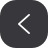被折叠的  条评论 为什么被折叠?到【灌水乐园】发言Jason~shen

¥1 ¥2 ¥4 ¥6 ¥10 ¥20获取中扫码支付点击重新获取扫码支付1.余额是钱包充值的虚拟货币，按照1:1的比例进行支付金额的抵扣。
2.余额无法直接购买下载，可以购买VIP、付费专栏及课程。余额充值